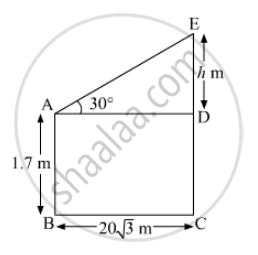Advertisement Remove all ads

# An Observer , 1.7 M Tall , is 20 √ 3 M Away from a Tower . the Angle of Elevation from the Eye of an Observer to the Top of Tower is 300 . Find the Height of the Tower. - Mathematics

Sum

An observer , 1.7 m tall , is 20 sqrt3  m away from a tower . The angle of elevation from the eye of an observer to the top of tower is 30. Find the height of the tower.

Advertisement Remove all ads

#### Solution

Let AB be the height of the observer and EC be the height of the tower.

Given:

AB=1.7 m ⇒ CD= 1.7 m

BC=20 sqrt3 m

Let ED be h m.In ∆ADE,

tan 30° = (ED)/(AD)

⇒ 1/sqrt3= h/(20sqrt3)

⇒ h=20 m

∴ EC=ED+DC=(h+1.7)m=21.7 m

Hence, the height of the tower is 21.7 m.

Is there an error in this question or solution?
Advertisement Remove all ads

#### APPEARS IN

RD Sharma Class 10 Maths
Chapter 12 Trigonometry
Q 10 | Page 41
Advertisement Remove all ads

#### Video TutorialsVIEW ALL 

Advertisement Remove all ads
Share
Notifications

View all notifications

Forgot password?
Course